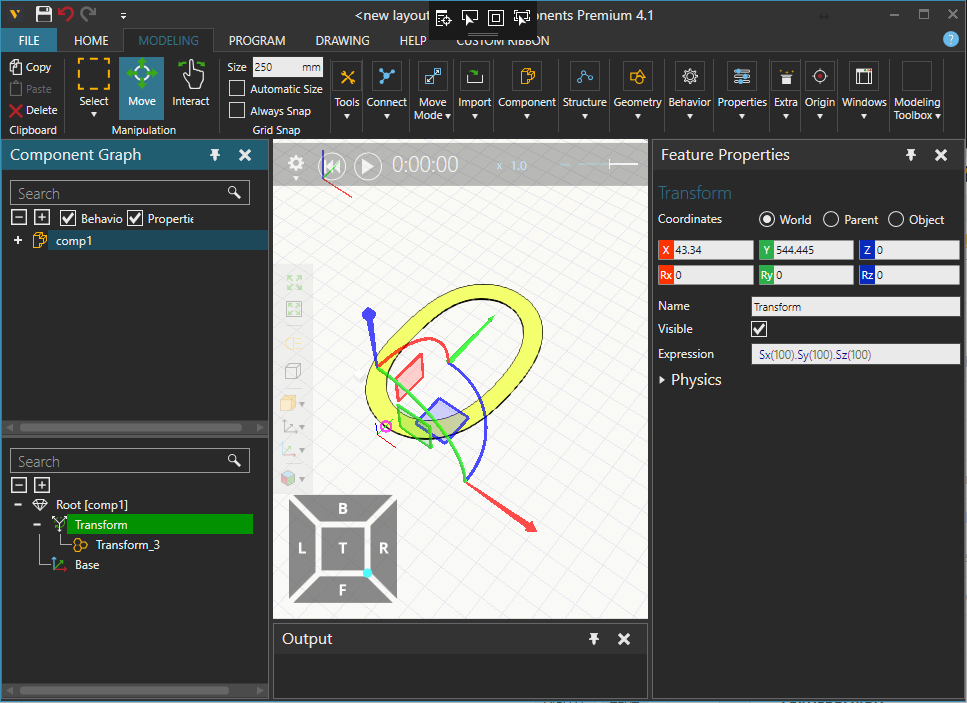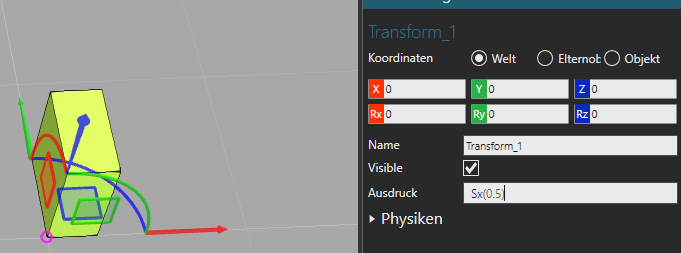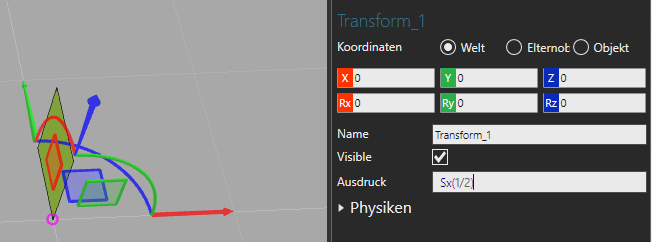# Scaling imported geometry

Hi,

Can Visual Components scale geometry that has been imported? I have a model from a customer that I want to use in a layout, however for some reason it is scaled at 1:100, i.e. I need it 100 times bigger. However apparently it is too physically large for Solidworks to scale up, so I was hoping VC could do it?

Hi,

For some formats you can affect scaling at import phase by playing with different units. For general case you can create a transform feature, put your geometries under that and then use scaling matrices in transform’s expression. So expression “Sx(100). Sy(100).Sz(100)” would scale up 100:1 child features in all X,Y and Z directions.-k

Hi,

I want to scale my geometry with a parameter (e.g. 20, 30,…), while the origin value is 100.

So I try the expression Sx(parameter/100). But the result was a zero thickness.What should I do?

My friend, what happens if you do “Sx(1.0/2)” ?

With this expression it works. So do I have to use a real instead of an integer?

The scaling parameter is a proportion of the original size in decimal format i.e. 0.5 = 50 % of the original and 1.5 = 150 % of the original size in the given axis direction.

Sx(0.5) is half the size in X-direction in the contained coordinates system.

If your “parameter” is an Integer property just do Sx(parameter/100.0)
Integer calculations result in an Integer value, while Integer divided by Real results in a Real value.

Okay, thanks guys!